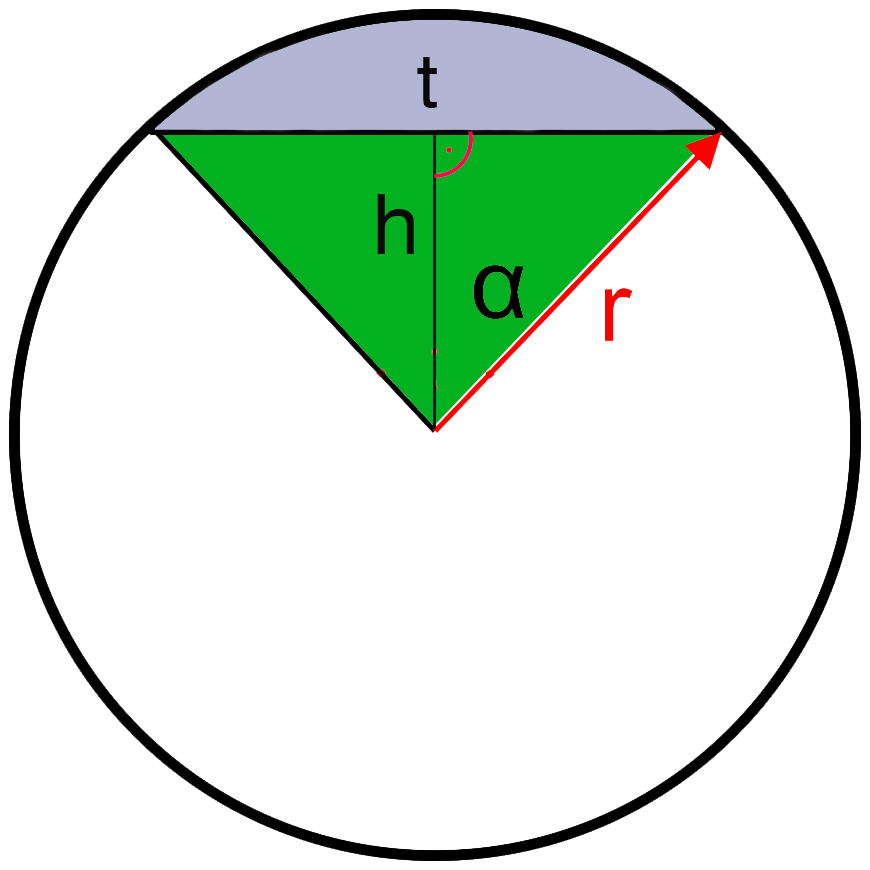﻿ Circle segment: - on line calculation, formula - FORMIAX

# Circle segment - circumference and surfaceC = 2πr. α / 360°

S=(πr2*360/α) - triangle surface area

C = circumference

S = surface area

α = uhol

t = chord

WHAT IS IT ?
Circle segment id the part of a circle made by a line and a connecting arc.

CALCULATION:

Enter unit e.g.: inch

Enter angle "α" in degrees

Round to number of decimals places

YOU MIGHT BE INTERESTED: